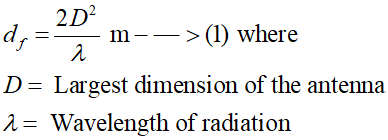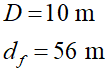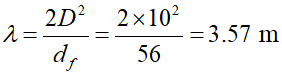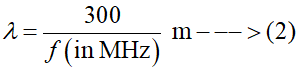# A transmitter is having a maximum dimention of 10 m and a far field distance of 56 m. What will be the frequency of transmission?

Question
1 views

A transmitter is having a maximum dimention of 10 m and a far field distance of 56 m. What will be the frequency of transmission?

check_circle

star
star
star
star
star
1 Rating
Step 1

For any antenna, the far-field distance (df) is given by the following equation:Step 2

In this case, the values of the maximum dimension of the antenna and the far-field distance are given asStep 3

Therefore, using equation (1), the wavelength of radiation will beThe frequency of transmission (f) and the wavelength of radiation are related by the following equation:...

### Want to see the full answer?

See Solution

#### Want to see this answer and more?

Solutions are written by subject experts who are available 24/7. Questions are typically answered within 1 hour.*

See Solution
*Response times may vary by subject and question.
Tagged in